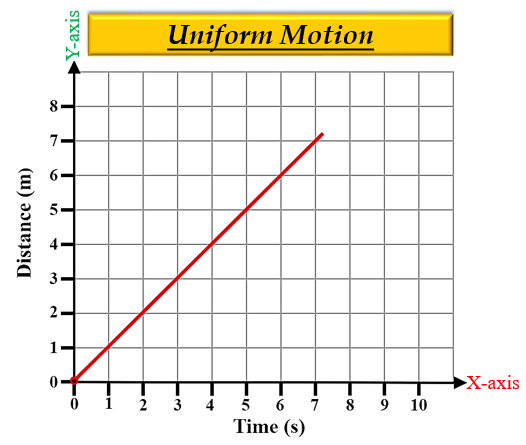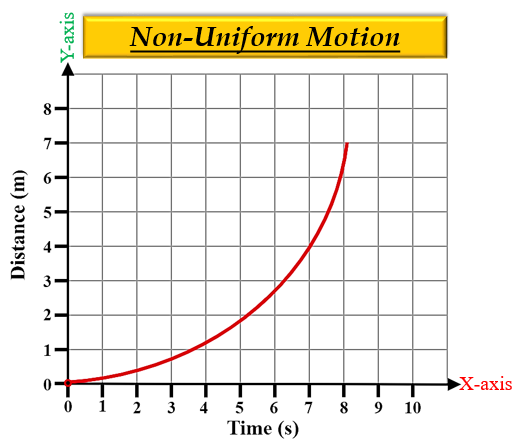# Draw the distance-time graph for the uniform and non-uniform motion of an object.

Distance-time graphs - These graphs give an idea of the distance travelled by the body with respect to time. They are plotted keeping distance along the y-axis (vertical line) and time along the x-axis (horizontal line).

There are  two types of distance-time graphs:

(I) Uniform motion- A body is said to be in uniform motion if it travels equal distances in equal intervals of time, no matter how small these time intervals may be. In other words, the distance travelled is directly proportional to time. Thus, a distance-time graph for uniform motion will be a straight line.(II) Non-Uniform motion- A body is said to be in a non-uniform motion if it travels unequal distances in equal intervals of time. In other words, motion is said to be accelerated. Thus, a distance-time graph for non-uniform motion will be a curved line.Updated on: 10-Oct-2022

305 Views